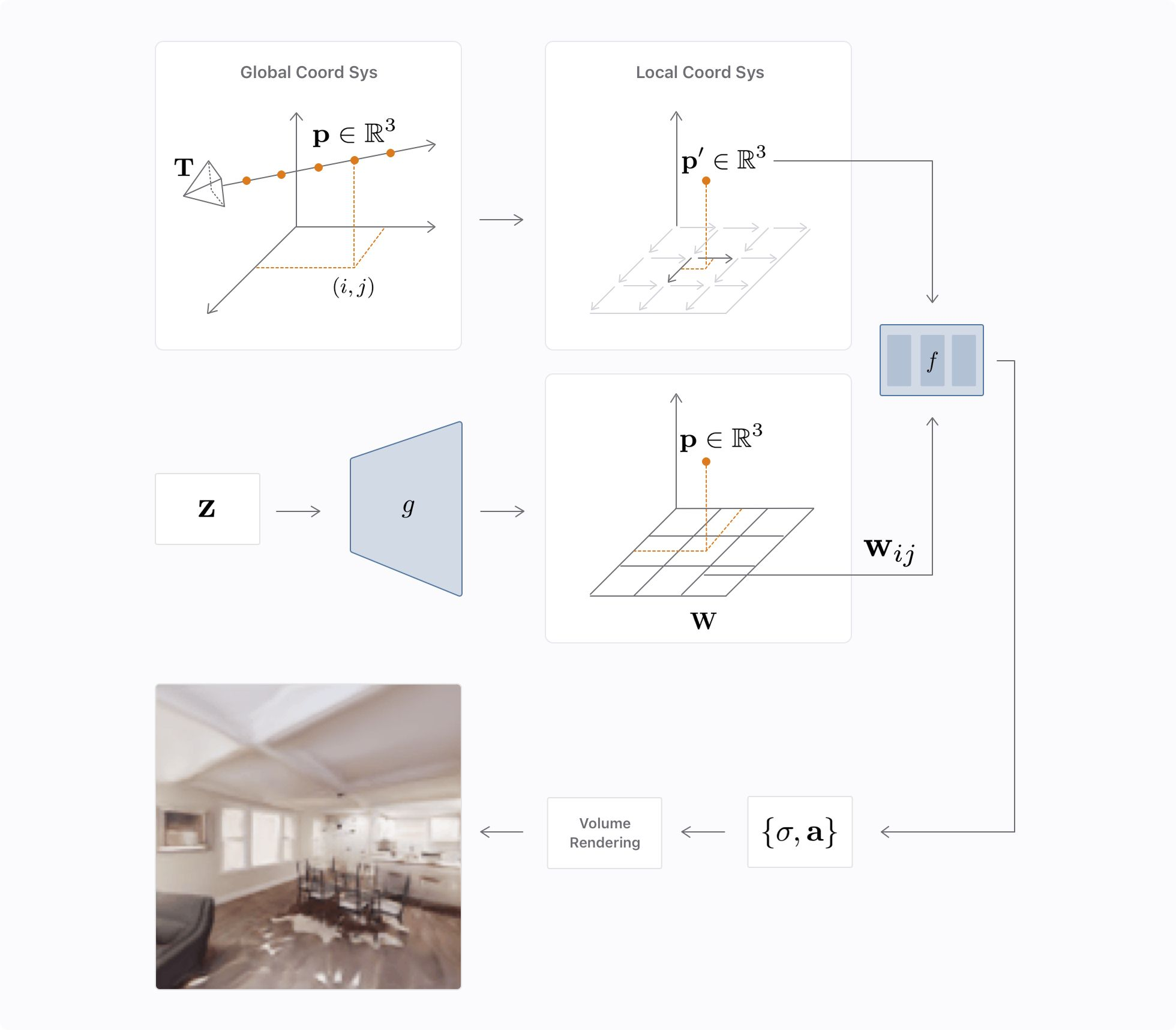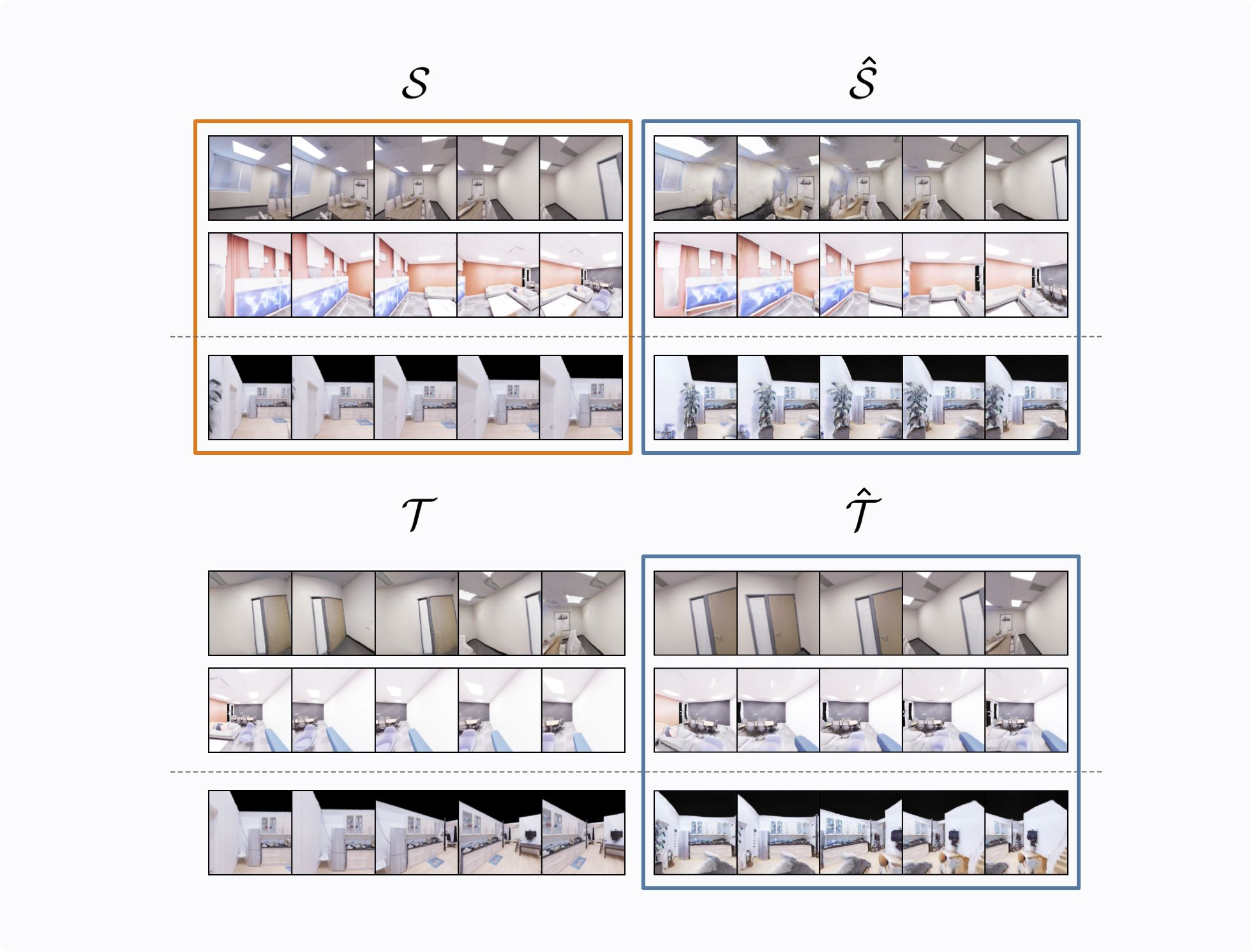People have an innate capability to understand the 3D visual world and make predictions about how the world could look from different points of view, even when relying on few visual observations. We have this spatial reasoning ability because of the rich mental models of the visual world we develop over time. These mental models can be interpreted as a prior belief over which configurations of the visual world are most likely to be observed. In this case, a prior is a probability distribution over the 3D visual world.

In this post we share our recent progress towards learning priors over the 3D visual world. In particular, we introduce Generative Scene Networks (GSN), models that are capable of learning a probability distribution over realistic and unconstrained indoor scenes. We follow an adversarial learning paradigm and represent scenes using radiance fields, jointly models geometry and appearance, while modeling view-dependent effects. This prevents our model from having to learn view consistency from data.

Learning a prior that effectively captures the true distribution over the 3D visual world—this is called a powerful prior—can have tremendous impact for a wide range of problems in machine learning. In particular, powerful priors of the 3D visual world can revolutionize the area of embodied AI where robotic agents are deployed in real world environments to solve tasks like localization, of the robotic agent within the world; navigation, where the agent goal is to navigate to a particular position of the environment; and, re-arrangement, where the goal is to re-arrange parts of the world to a given goal configuration.

The objective in GSN is to learn a generative model of scenes given a collection of real scene images. We propose following an adversarial learning game paradigm. In this paradigm, two players (a generator and a discriminator) compete against each other. The generator’s task is to generate scenes and render images from them using camera poses sampled from an empirical distribution. On the other hand, the discriminator takes images rendered in the generator and tries to predict whether they belong to the empirical distribution of real scene images or not.

In GSN, scenes are represented using radiance fields, a functional representation that jointly models geometry and appearance, and is able to model view-dependent effects. A radiance field is implemented as a parametric function $f_\theta(\mathbf{p}, \mathbf{d})$ a multilayer perceptron (MLP), which is a fully connected neural network with multiple layers of features, that takes as input a 3D point $\mathbf{p}$ and a camera direction $\mathbf{d}$ and predicts a density scalar and an RGB color vector. Typically, the parameters $\theta$ of the MLP are learned by minimizing an MSE reconstruction loss with regards to a dense capture of views of the scene. In our paradigm, similar to GRAF, parameters $\theta$ are learned via the adversarial game between the generator and discriminator.

At a high level, in GSN we decompose the parameters $\theta = \theta_f + \mathbf{w}$ of the radiance field into a set of base parameters $\theta_f$ (the parameters of the radiance field MLP) and a latent vector $\mathbf{w}$ that is predicted by the generator. In this setting, $\mathbf{w}$ is used to perform a feature-wise linear modulation of the activations in $f$ which is often defined as “conditioning."

## Representing a Scene with Local Radiance Fields

Instead of using a single vector $\mathbf{w}$ for conditioning, we propose to distribute $\mathbf{w}$ into a 2D spatial grid that is interpreted as a latent floorplan representation. Intuitively, the decomposition of $\mathbf{w}$ into a spatial grid amounts to modeling a scene with multiple local radiance fields (with one radiance field per $\mathbf{w}_{ij}$ vector on the grid), that work collectively to produce a scene-level radiance field.Figure 2: The architecture of the GSN generator. We decompose the generator into two sub-modules, a global generator ggg and a local radiance field function fff.

In Figure 2 we show the architecture for the generator in GSN. We sample a latent code $\mathbf{z} ∼ p_z$ that is fed to our global generator $g$ producing a local latent grid $\mathbf{W}$. This local latent grid $\mathbf{W}$ conceptually represents a latent scene floor plan and is used for locally conditioning a radiance field $f$ from which images are rendered via volumetric rendering. For a given point $\mathbf{p}$ expressed in a global coordinate system to be rendered, we sample $\mathbf{W}$ at the location $(i, j)$, given by $\mathbf{p}$ resulting in $\mathbf{w}_{ij}$. In turn, $f$ takes as input $\mathbf{p}'$, which results from expressing $\mathbf{p}$ relative to the local coordinate system of $\mathbf{w}_{ij}$.

At its essence, GSN can be interpreted as a Generative Adversarial Network (GAN) for 3D scenes instead of single images, where the generator has a particular structure that allows it to generate radiance fields and the discriminator is a standard 2D convolutional discriminator as used in GANs for images. As a result, training GSN is not harder than training any other GAN architecture, and GSN can leverage the latest advances for increasing training stability.

## View Synthesis

An interesting application of GSN is view synthesis, which showcases the abilities of GSN to act as mental model of the world that can be used to complete a scene given partial observations. In this application, we are given a set $\mathcal{S}$ of source views and camera poses and we want to predict views at given target camera poses $\mathcal{T}$. To approach this application, we take a trained GSN generator and perform inversion to find a latent code $\mathbf{z}$ from the prior that minimizes a reconstruction loss with respect to $\mathcal{S}$.

The result of the inversion is denoted as $\hat{\mathcal{S}}$. Once this latent code $\mathbf{z}$ is obtained, we simply render the resulting scene-level radiance field from the target camera poses. We observe that GSN performs exceptionally well for this task even if it was not explicitly designed for it. Results in Figure 4 show how our model is able to correctly predict parts of the scene that were not observed in the source views.Figure 4: Qualitative view synthesis results on the Replica Dataset. The frames highlighted in orange are used by GSN to perform inversion. The frames highlighted in blue are predicted by the model.

In Figure 4 we show qualitative view synthesis results on Replica Dataset. Given source views $\mathcal{S}$, we invert GSN to obtain a local latent code grid $\hat{\mathcal{W}}$ , which is then used both to reconstruct $\mathcal{S}$, denoted as $\hat{\mathcal{S}}$, and also to predict target views $\mathcal{T}$ (given their camera poses) which are denoted as $\hat{\mathcal{T}}$ . Each row corresponds to a different set of source views $\mathcal{S}$. The top three rows are scenes from the training set, and the bottom three rows are scenes in a held out test set.

We observe that inverting GSN provides good scene completion. Notice how our model correctly predicts the existence of the door in the first scene—the first row of Figure 4—by observing a very small portion of it in the source views $\mathcal{S}$. In addition, we notice that for scenes unseen during training—the third row of Figure 4—the model performs reasonably if the training set contains similar samples.

# Conclusions

In this post we discussed GSN, a generative model for unconstrained 3D scenes that represents scenes via radiance fields. In the GSN model, the scene radiance field is decomposed into many local radiance fields that collectively model the scene. We learned that GSN can be used for different downstream tasks like view synthesis or spatial scene editing. We are excited about the next steps of this research area and its applications on embodied machine learning tasks.

If this post and area of research are interesting to you check out opportunities on our team here.

# Acknowledgments

Many people contributed to this work including Miguel Angel Bautista Martin, Terrance DeVries, Nitish Srivastava, and Josh Susskind.

# Resources

Download the two datasets that were used to train. the "Generative Scene Networks" model.

# References

Chan, Eric R., et al. "pi-gan: Periodic implicit generative adversarial networks for 3d-aware image synthesis." Proceedings of the IEEE/CVF Conference on Computer Vision and Pattern Recognition. 2021. [link].

Ha, David, and Jürgen Schmidhuber. "World models." arXiv preprint arXiv:1803.10122 (2018). [link].

Mildenhall, Ben, et al. "Nerf: Representing scenes as neural radiance fields for view synthesis." European conference on computer vision. Springer, Cham, 2020. [link].

Perez, Ethan, et al. "Film: Visual reasoning with a general conditioning layer." Proceedings of the AAAI Conference on Artificial Intelligence. Vol. 32. No. 1. 2018. [link].

Schwarz, Katja, et al. "GRAF: Generative Radiance Fields for 3D-Aware Image Synthesis." Advances in Neural Information Processing Systems 33 (2020). [link].

Straub, Julian, et al. “The Replica dataset: A digital replica of indoor spaces.” arXiv preprint arXiv:1906.05797 (2019). [link].

Wang, Qianqian, et al. "Ibrnet: Learning multi-view image-based rendering." Proceedings of the IEEE/CVF Conference on Computer Vision and Pattern Recognition. 2021. [link].

Yu, Alex, et al. "pixelnerf: Neural radiance fields from one or few images." Proceedings of the IEEE/CVF Conference on Computer Vision and Pattern Recognition. 2021. [link].